# An order-type existence theorem and applications to periodic problems

## Abstract

Based on the fixed point index and partial order method, one new order-type existence theorem concerning cone expansion and compression is established. As applications, we present sufficient existence conditions for the first- and second-order periodic problems.

MSC:34B15.

## 1 Introduction and preliminaries

Let X, Y be real Banach spaces. Consider a linear mapping $L:domL\subset X\to Y$ and a nonlinear operator $N:X\to Y$. Here we assume that L is a Fredholm operator of index zero, that is, ImL is closed and $dim KerL=codim ImL<\mathrm{\infty }$. Then the solvability of the operator equation

$Lx=Nx$

has been studied by many researchers in the literature; see  and the references therein. In , Cremins established a fixed point index for A-proper semilinear operators defined on cones which includes and improves the results in [5, 8, 9]. Using the fixed point index and the concept of a quasi-normal cone introduced in , Cremins established a norm-type existence theorem concerning cone expansion and compression in , which generalizes some corresponding results contained in .

In this paper, we will use the properties of the fixed point index in  and partial order to present a new order-type existence theorem concerning cone expansion and compression which extends the corresponding results in . We recall that a partial order in X induced by a cone $K\subset X$ is defined by

$x\le y\phantom{\rule{1em}{0ex}}⟺\phantom{\rule{1em}{0ex}}y-x\in K.$

As applications, we study the first- and second-order periodic boundary problems and obtain new existence results. During the last few decades, periodic boundary value problems have been studied by many researchers in the literature; see, for example,  and the references therein. Our new results improve those contained in [13, 18].

Next we recall some notations and results which will be needed in this paper. Let X and Y be Banach spaces, D be a linear subspace of X, $\left\{{X}_{n}\right\}\subset D$ and $\left\{{Y}_{n}\right\}\subset Y$ be the sequences of oriented finite dimensional subspaces such that ${Q}_{n}y\to y$ in Y for every y and dist$\left(x,{X}_{n}\right)\to 0$ for every $x\in D$, where ${Q}_{n}:Y\to {Y}_{n}$ and ${P}_{n}:X\to {X}_{n}$ are sequences of continuous linear projections. The projection scheme $\mathrm{\Gamma }=\left\{{X}_{n},{Y}_{n},{P}_{n},{Q}_{n}\right\}$ is then said to be admissible for maps from $D\subset X$ to Y. A map $T:D\subset X\to Y$ is called approximation-proper (abbreviated A-proper) at a point $y\in Y$ with respect to an admissible scheme Γ if ${T}_{n}\equiv {Q}_{n}T{|}_{D\cap {X}_{n}}$ is continuous for each $n\in \mathbb{N}$ and whenever $\left\{{x}_{{n}_{j}}:{x}_{{n}_{j}}\in D\cap {X}_{{n}_{j}}\right\}$ is bounded with ${T}_{{n}_{j}}{x}_{{n}_{j}}\to y$, then there exists a subsequence $\left\{{x}_{{n}_{{j}_{k}}}\right\}$ such that ${x}_{{n}_{{j}_{k}}}\to x\in D$ and $Tx=y$. T is simply called A-proper if it is A-proper at all points of Y. $L:domL\subset X\to Y$ is a Fredholm operator of index zero if ImL is closed and $dim KerL=codim ImL<\mathrm{\infty }$. As a consequence of this property, X and Y may be expressed as direct sums; $X={X}_{0}⨁{X}_{1}$, $Y={Y}_{0}\oplus {Y}_{1}$ with continuous linear projections $P:X\to KerL={X}_{0}$ and $Q:Y\to {Y}_{0}$. The restriction of L to $domL\cap {X}_{1}$, denoted ${L}_{1}$, is a bijection onto $ImL={Y}_{1}$ with continuous inverse ${L}_{1}^{-1}:{Y}_{1}\to domL\cap {X}_{1}$. Since ${X}_{0}$ and ${Y}_{0}$ have the same finite dimension, there exists a continuous bijection $J:{Y}_{0}\to {X}_{0}$. Let $H=L+{J}^{-1}P$, then $H:domL\subset X\to Y$ is a linear bijection with bounded inverse. Let K be a cone in a Banach space X. Then ${K}_{1}=H\left(K\cap domL\right)$ is a cone in Y. In , Petryshyn has shown that an admissible scheme ${\mathrm{\Gamma }}_{L}$ can be constructed such that L is A-proper with respect to ${\mathrm{\Gamma }}_{L}$. The following properties of the fixed point index ${ind}_{K}$ and two lemmas can be found in .

Proposition 1.1 Let $\mathrm{\Omega }\subset X$ be open and bounded and $\partial {\mathrm{\Omega }}_{K}=\partial \mathrm{\Omega }\cap K$. Assume that ${Q}_{n}{K}_{1}\subset {K}_{1}$, $P+JQN+{L}_{1}^{-1}\left(I-Q\right)N$ maps K to K, and $Lx\ne Nx$ on $\partial {\mathrm{\Omega }}_{K}$.

(P1) (Existence property) If ${ind}_{K}\left(\left[L,N\right],\mathrm{\Omega }\right)\ne \left\{0\right\}$, then there exists $x\in {\mathrm{\Omega }}_{K}$ such that $Lx=Nx$.

(P2) (Normality) If ${x}_{0}\in {\mathrm{\Omega }}_{K}$, then ${ind}_{K}\left(\left[L,-{J}^{-1}P+{\stackrel{ˆ}{y}}_{0}\right],\mathrm{\Omega }\right)=\left\{1\right\}$, where ${\stackrel{ˆ}{y}}_{0}=H{x}_{0}$ and ${\stackrel{ˆ}{y}}_{0}\left(y\right)={y}_{0}$ for every $y\in H{\mathrm{\Omega }}_{K}$.

(P3) (Additivity) If $Lx\ne Nx$ for $x\in {\overline{\mathrm{\Omega }}}_{K}\mathrm{\setminus }\left({\mathrm{\Omega }}_{1}\cup {\mathrm{\Omega }}_{2}\right)$, where ${\mathrm{\Omega }}_{1}$ and ${\mathrm{\Omega }}_{2}$ are disjoint relatively open subsets of ${\mathrm{\Omega }}_{K}$, then

${ind}_{K}\left(\left[L,N\right],\mathrm{\Omega }\right)\subseteq {ind}_{K}\left(\left[L,N\right],{\mathrm{\Omega }}_{1}\right)+{ind}_{K}\left(\left[L,N\right],{\mathrm{\Omega }}_{2}\right)$

with equality if either of indices on the right is a singleton.

(P4) (Homotopy invariance) If $L-N\left(\lambda ,x\right)$ is an A-proper homotopy on ${\mathrm{\Omega }}_{K}$ for $\lambda \in \left[0,1\right]$ and $\left(N\left(\lambda ,x\right)+{J}^{-1}P\right){H}^{-1}:{K}_{1}\to {K}_{1}$ and $\theta \notin \left(L-N\left(\lambda ,x\right)\right)\left(domL\cap \partial {\mathrm{\Omega }}_{K}\right)$ for $\lambda \in \left[0,1\right]$, then ${ind}_{K}\left(\left[L,N\left(\lambda ,x\right)\right],\mathrm{\Omega }\right)={ind}_{{K}_{1}}\left({T}_{\lambda },U\right)$ is independent of $\lambda \in \left[0,1\right]$, where ${T}_{\lambda }=\left(N\left(\lambda ,x\right)+{J}^{-1}P\right){H}^{-1}$.

Lemma 1.1 If $L:domL\to Y$ is Fredholm of index zero, Ω is an open bounded set and ${\mathrm{\Omega }}_{K}\cap domL\ne \mathrm{\varnothing }$, $\theta \in \mathrm{\Omega }\subset X$. Let $L-\lambda N$ be A-proper for $\lambda \in \left[0,1\right]$. Assume that N is bounded and $P+JQN+{L}_{1}^{-1}\left(I-Q\right)N$ maps K to K. If $Lx\ne \mu Nx-\left(1-\mu \right){J}^{-1}Px$ on $\partial {\mathrm{\Omega }}_{K}$ for $\mu \in \left[0,1\right]$, then

${ind}_{K}\left(\left[L,N\right],\mathrm{\Omega }\right)=\left\{1\right\}.$

Lemma 1.2 If $L:domL\to Y$ is Fredholm of index zero, Ω is an open bounded set and ${\mathrm{\Omega }}_{K}\cap domL\ne \mathrm{\varnothing }$. Let $L-\lambda N$ be A-proper for $\lambda \in \left[0,1\right]$. Assume that N is bounded and $P+JQN+{L}_{1}^{-1}\left(I-Q\right)N$ maps K to K. If there exists $e\in {K}_{1}\mathrm{\setminus }\left\{\theta \right\}$ such that

$Lx-Nx\ne \mu e,$

for every $x\in \partial {\mathrm{\Omega }}_{K}$ and all $\mu \ge 0$, then

${ind}_{K}\left(\left[L,N\right],\mathrm{\Omega }\right)=\left\{0\right\}.$

## 2 An abstract result

We will establish an abstract existence theorem concerning cone expansion and compression of order type, which reads as follows.

Theorem 2.1 If $L:domL\to Y$ is Fredholm of index zero, let $L-\lambda N$ be A-proper for $\lambda \in \left[0,1\right]$. Assume that N is bounded and $P+JQN+{L}_{1}^{-1}\left(I-Q\right)N$ maps K to K. Suppose further that ${\mathrm{\Omega }}_{1}$ and ${\mathrm{\Omega }}_{2}$ are two bounded open sets in X such that $\theta \in {\mathrm{\Omega }}_{1}\subset {\overline{\mathrm{\Omega }}}_{1}\subset {\mathrm{\Omega }}_{2}$, ${\mathrm{\Omega }}_{1}\cap K\cap domL\ne \mathrm{\varnothing }$ and ${\mathrm{\Omega }}_{2}\cap K\cap domL\ne \mathrm{\varnothing }$. If one of the following two conditions is satisfied:

(C1) $\left(P+JQN\right)x+{L}_{1}^{-1}\left(I-Q\right)Nx\ngeqq x$ for all $x\in \partial {\mathrm{\Omega }}_{1}\cap K$ and $\left(P+JQN\right)x+{L}_{1}^{-1}\left(I-Q\right)Nx\nleqq x$ for all $x\in \partial {\mathrm{\Omega }}_{2}\cap K$;

(C2) $\left(P+JQN\right)x+{L}_{1}^{-1}\left(I-Q\right)Nx\nleqq x$ for all $x\in \partial {\mathrm{\Omega }}_{1}\cap K$ and $\left(P+JQN\right)x+{L}_{1}^{-1}\left(I-Q\right)Nx\ngeqq x$ for all $x\in \partial {\mathrm{\Omega }}_{2}\cap K$.

Then there exists $x\in \left({\overline{\mathrm{\Omega }}}_{2}\mathrm{\setminus }{\mathrm{\Omega }}_{1}\right)\cap K$ such that $Lx=Nx$.

Proof We assume that (C1) is satisfied. First we show that

(2.1)

In fact, otherwise, there exist ${x}_{1}\in \partial {\mathrm{\Omega }}_{1}\cap K$ and ${\mu }_{1}\in \left[0,1\right]$ such that

$L{x}_{1}={\mu }_{1}N{x}_{1}-\left(1-{\mu }_{1}\right){J}^{-1}P{x}_{1},$

then we obtain

$\left(L+{J}^{-1}P\right){x}_{1}={\mu }_{1}\left(N+{J}^{-1}P\right){x}_{1}.$

Therefore,

$\begin{array}{rcl}{x}_{1}& =& {\mu }_{1}{\left(L+{J}^{-1}P\right)}^{-1}\left(N+{J}^{-1}P\right){x}_{1}\\ =& {\mu }_{1}\left[\left(P+JQN\right){x}_{1}+{L}_{1}^{-1}\left(I-Q\right)N{x}_{1}\right]\\ \le & \left(P+JQN\right){x}_{1}+{L}_{1}^{-1}\left(I-Q\right)N{x}_{1},\end{array}$

which contradicts condition (C1). From (2.1) and Lemma 1.1, we have

${ind}_{K}\left(\left[L,N\right],{\mathrm{\Omega }}_{1}\right)=\left\{1\right\}.$
(2.2)

Choosing an arbitrary $e\in {K}_{1}\mathrm{\setminus }\left\{\theta \right\}$, next we prove that

$Lx-Nx\ne \mu e.$
(2.3)

In fact, otherwise, there exist ${x}_{2}\in \partial {\mathrm{\Omega }}_{2}\cap K$ and ${\mu }_{2}\ge 0$ such that

$L{x}_{2}-N{x}_{2}={\mu }_{2}e,$

then we obtain

$\left(L+{J}^{-1}P\right){x}_{2}=\left(N+{J}^{-1}P\right){x}_{2}+{\mu }_{2}e{\ge }_{1}\left(N+{J}^{-1}P\right){x}_{2},$

in which the partial order is induced by the cone ${K}_{1}$ in Y. So,

${x}_{2}\ge {\left(L+{J}^{-1}P\right)}^{-1}\left(N+{J}^{-1}P\right){x}_{2}=\left(P+JQN\right){x}_{2}+{L}_{1}^{-1}\left(I-Q\right)N{x}_{2},$

which is a contradiction to condition (C1). Hence (2.3) holds, and then by Lemma 1.2, we have

${ind}_{K}\left(\left[L,N\right],{\mathrm{\Omega }}_{2}\right)=\left\{0\right\}.$
(2.4)

It follows therefore from (2.2), (2.4) and the additivity property (P3) of Proposition 1.1 that

$\begin{array}{rcl}{ind}_{K}\left(\left[L,N\right],{\mathrm{\Omega }}_{2}\mathrm{\setminus }{\mathrm{\Omega }}_{1}\right)& =& {ind}_{K}\left(\left[L,N\right],{\mathrm{\Omega }}_{2}\right)-{ind}_{K}\left(\left[L,N\right],{\mathrm{\Omega }}_{1}\right)\\ =& \left\{0\right\}-\left\{1\right\}\\ =& \left\{-1\right\}.\end{array}$
(2.5)

Since the index is nonzero, the existence property (P1) of Proposition 1.1 implies that there exists $x\in \left({\overline{\mathrm{\Omega }}}_{2}\mathrm{\setminus }{\mathrm{\Omega }}_{1}\right)\cap K$ such that $Lx=Nx$.

Similarly, when (C2) is satisfied, instead of (2.2), (2.4) and (2.5), we have

${ind}_{K}\left(\left[L,N\right],{\mathrm{\Omega }}_{1}\right)=\left\{0\right\},\phantom{\rule{2em}{0ex}}{ind}_{K}\left(\left[L,N\right],{\mathrm{\Omega }}_{2}\right)=\left\{1\right\},$

and therefore

${ind}_{K}\left(\left[L,N\right],{\mathrm{\Omega }}_{2}\mathrm{\setminus }{\mathrm{\Omega }}_{1}\right)=\left\{1\right\}.$

Also, we can assert that there exists $x\in \left({\overline{\mathrm{\Omega }}}_{2}\mathrm{\setminus }{\mathrm{\Omega }}_{1}\right)\cap K$ such that $Lx=Nx$. □

## 3 Applications

### 3.1 First-order periodic boundary value problems

We consider the following first-order periodic boundary value problem:

$\left\{\begin{array}{c}{x}^{\prime }\left(t\right)=f\left(t,x\left(t\right)\right),\phantom{\rule{1em}{0ex}}t\in \left(0,1\right),\hfill \\ x\left(0\right)=x\left(1\right),\hfill \end{array}$
(3.1)

where $f:\left[0,1\right]×\left[0,+\mathrm{\infty }\right)\to \mathbb{R}$ is continuous and $f\left(0,x\right)=f\left(1,x\right)$ for all $x\in \mathbb{R}$.

Consider the Banach spaces $X=Y=C\left[0,1\right]$ endowed with the norm $\parallel x\parallel ={max}_{t\in \left[0,1\right]}|x\left(t\right)|$. Define the cone K in X by

$K=\left\{x\in X:x\left(t\right)\ge 0,t\in \left[0,1\right]\right\}.$

Let L be the linear operator from $domL\subset X$ to Y with

$domL=\left\{x\in X:{x}^{\prime }\in C\left[0,1\right],x\left(0\right)=x\left(1\right)\right\},$

and

$Lx\left(t\right)={x}^{\prime }\left(t\right),\phantom{\rule{1em}{0ex}}x\in domL,t\in \left[0,1\right].$

Let us define $N:X\to Y$ by

$Nx\left(t\right)=f\left(t,x\left(t\right)\right),\phantom{\rule{1em}{0ex}}t\in \left[0,1\right].$

Then (3.1) is equivalent to the equation

$Lx=Nx.$

It is obvious that L is a Fredholm operator of index zero with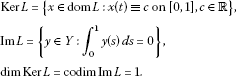Next we define the projections $P:X\to X$, $Q:Y\to Y$ by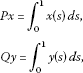and the isomorphism $J:ImQ\to ImP$ as $Jy=y$. Note that for $y\in ImL$, the inverse operator

${L}_{1}^{-1}:ImL\to domL\cap KerP$

of

$L{|}_{domL\cap KerP}:domL\cap KerP\to ImL$

is given by

$\left({L}_{1}^{-1}y\right)\left(t\right)={\int }_{0}^{1}K\left(t,s\right)y\left(s\right)\phantom{\rule{0.2em}{0ex}}ds,$

where

$K\left(t,s\right)=\left\{\begin{array}{cc}s+1,\hfill & 0\le s

Set

$G\left(t,s\right)=1+K\left(t,s\right)-{\int }_{0}^{1}K\left(t,s\right)\phantom{\rule{0.2em}{0ex}}ds.$

We can verify that

$G\left(t,s\right)=\left\{\begin{array}{cc}\frac{3}{2}-\left(t-s\right),\hfill & 0\le s

and

$\frac{1}{2}\le G\left(t,s\right)\le \frac{3}{2},\phantom{\rule{1em}{0ex}}t,s\in \left[0,1\right].$

To state the existence result, we introduce two conditions:

(H1) $f\left(t,b\right)<0$ for all $t\in \left[0,1\right]$,

(H2) $f\left(t,x\right)>0$ for all $\left(t,x\right)\in \left[0,1\right]×\left[0,a\right]$.

Theorem 3.1 Assume that there exist two positive numbers $0 such that (H1), (H2) and

(H3) $f\left(t,x\right)\ge -\frac{2}{3}x$ for all $\left(t,x\right)\in \left[0,1\right]×\left[0,b\right]$

hold. Then (3.1) has at least one positive periodic solution ${x}^{\ast }\in K$ with $a\le \parallel {x}^{\ast }\parallel \le b$.

Proof First, we note that L, as defined, is Fredholm of index zero, ${L}_{1}^{-1}$ is compact by the Arzela-Ascoli theorem and thus $L-\lambda N$ is A-proper for $\lambda \in \left[0,1\right]$ by [, Lemma 2(a)].

For each $x\in K$, then by condition (H3),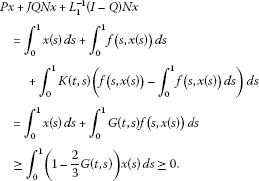Thus $\left(P+JQN+{L}_{1}^{-1}\left(I-Q\right)N\right)\left(K\right)\subset K$.

Let

${\mathrm{\Omega }}_{1}=\left\{x\in X:\parallel x\parallel

Clearly, ${\mathrm{\Omega }}_{1}$ and ${\mathrm{\Omega }}_{2}$ are bounded open sets and

$\theta \in {\mathrm{\Omega }}_{1}\subset {\overline{\mathrm{\Omega }}}_{1}\subset {\mathrm{\Omega }}_{2}.$

We now show that

(3.2)

In fact, if there exists ${x}_{3}\in \partial {\mathrm{\Omega }}_{2}\cap K$ such that

$\left(P+JQN\right){x}_{3}+{L}_{1}^{-1}\left(I-Q\right)N{x}_{3}\ge {x}_{3}.$

Then

${x}_{3}^{\prime }\left(t\right)\le f\left(t,{x}_{3}\left(t\right)\right),\phantom{\rule{1em}{0ex}}t\in \left[0,1\right].$

Let ${t}_{1}\in \left[0,1\right]$ be such that ${x}_{3}\left({t}_{1}\right)=b$. Clearly, the function ${x}_{3}^{2}$ attains a maximum on $\left[0,1\right]$ at $t={t}_{1}$. Therefore $2{x}_{3}\left({t}_{1}\right){x}_{3}^{\prime }\left({t}_{1}\right)=0$. As a consequence,

$0=2b{x}_{3}^{\prime }\left({t}_{1}\right)\le 2bf\left({t}_{1},{x}_{3}\left({t}_{1}\right)\right)=2bf\left({t}_{1},b\right),$

which is a contradiction to (H1). Therefore (3.2) holds.

On the other hand, we claim that

(3.3)

In fact, if not, there exists ${x}_{4}\in \partial {\mathrm{\Omega }}_{1}\cap K$ such that

$\left(P+JQN\right){x}_{4}+{L}_{1}^{-1}\left(I-Q\right)N{x}_{4}\le {x}_{4}.$

For any ${x}_{4}\in \partial {\mathrm{\Omega }}_{1}\cap K$, we have $\parallel {x}_{4}\parallel =a$, then $0\le {x}_{4}\left(t\right)\le a$ for $t\in \left[0,1\right]$. By condition (H2), we have

which is a contradiction. As a result, (3.3) is verified.

It follows from (3.2), (3.3) and Theorem 2.1 that there exists ${x}^{\ast }\in K\cap \left({\overline{\mathrm{\Omega }}}_{2}\mathrm{\setminus }{\mathrm{\Omega }}_{1}\right)$ such that $L{x}^{\ast }=N{x}^{\ast }$ with $a\le \parallel {x}^{\ast }\parallel \le b$. □

Remark 3.1 In , the following condition is required instead of (H2):

(H) there exist $a\in \left(0,b\right)$, ${t}_{0}\in \left[0,1\right]$, $r\in \left(0,1\right]$, and continuous functions $g:\left[0,1\right]\to \left[0,\mathrm{\infty }\right)$, $h:\left(0,a\right]\to \left[0,\mathrm{\infty }\right)$ such that $f\left(t,x\right)\ge g\left(t\right)h\left(x\right)$ for all $t\in \left[0,1\right]$ and $x\in \left(0,a\right]$, $h\left(x\right)/{x}^{r}$ is nonincreasing on $\left(0,a\right]$ with

$\frac{h\left(a\right)}{{2}^{r-1}}{\int }_{0}^{1}G\left({t}_{0},s\right)g\left(s\right)\phantom{\rule{0.2em}{0ex}}ds\ge a.$

Obviously, our condition (H2) is much weaker and less strict compared with (H). Moreover, (H2) is easier to check than (H). So, our result generalizes and improves [, Theorem 5].

Remark 3.2 From the proof of Theorem 3.1, we can see that condition (H2) can be replaced by one of the following two relatively weaker conditions:

(${\mathrm{H}}_{2}^{\ast }$) $f\left(t,x\right)\ge 0$ for all $\left(t,x\right)\in \left[0,1\right]×\left[0,a\right]$ and $f\left(t,\cdot \right)$ is positive for almost everywhere on $\left[0,a\right]$.

(${\mathrm{H}}_{2}^{\ast \ast }$) ${lim}_{x\to {0}^{+}}{min}_{t\in \left[0,1\right]}f\left(t,x\right)>0$.

Remark 3.3 Finally in this section, we note that conditions (H1) and (H2) can be replaced by the following asymptotic conditions:

(${\mathrm{H}}_{1}^{\prime }$) ${lim}_{x\to +\mathrm{\infty }}\frac{f\left(t,x\right)}{x}<0$ uniformly for t;

(${\mathrm{H}}_{2}^{\prime }$) ${lim}_{x\to {0}^{+}}\frac{f\left(t,x\right)}{x}>0$ uniformly for t.

Example 3.1 Let the nonlinearity in (3.1) be

$f\left(t,x\right)=c\left(t\right){x}^{\alpha }+\mu d\left(t\right){x}^{\beta }-kx,$

where $0<\alpha <1<\beta$, $c\left(t\right),d\left(t\right)\in C\left[0,1\right]$ are positive 1-periodic functions, $k\in \left(0,2/3\right)$ and $\mu >0$ is a positive parameter. Then (3.1) has at least one positive 1-periodic solution for each $0<\mu <{\mu }^{\ast }$, here ${\mu }^{\ast }$ is some positive constant.

Proof We will apply Theorem 3.1 with $f\left(t,x\right)=c\left(t\right){x}^{\alpha }+\mu d\left(t\right){x}^{\beta }-kx$. Since $k\in \left(0,2/3\right)$, it is easy to see that (H3) holds. Set

$T\left(x\right)=\frac{kx-{c}^{\ast }{x}^{\alpha }}{{d}^{\ast }{x}^{\beta }},$

where

${c}^{\ast }=\underset{t}{max}c\left(t\right),\phantom{\rule{2em}{0ex}}{d}^{\ast }=\underset{t}{max}d\left(t\right).$

Since $0<\alpha <1<\beta$, we have

$T\left({0}^{+}\right)=-\mathrm{\infty },\phantom{\rule{2em}{0ex}}T\left(+\mathrm{\infty }\right)=0.$

One may easily see that there exists $b>0$ such that

$T\left(b\right)=\frac{kb-{c}^{\ast }{b}^{\alpha }}{{d}^{\ast }{b}^{\beta }}=\underset{x>0}{sup}T\left(x\right)>0.$

Let

${\mu }^{\ast }=\frac{kb-{c}^{\ast }{b}^{\alpha }}{{d}^{\ast }{b}^{\beta }}.$

Then, for each $\mu \in \left(0,{\mu }^{\ast }\right)$, we have

$\begin{array}{rcl}f\left(t,b\right)& =& c\left(t\right){b}^{\alpha }+\mu d\left(t\right){b}^{\beta }-kb\\ <& {c}^{\ast }{b}^{\alpha }+{\mu }^{\ast }{d}^{\ast }{b}^{\beta }-kb\\ =& 0,\end{array}$

which implies that (H1) holds.

On the other hand, we have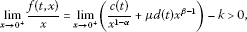which implies that (${\mathrm{H}}_{2}^{\prime }$) holds. Now we have the desired result. □

### 3.2 Second-order periodic boundary value problems

Let $f:\left[0,1\right]×\left[0,+\mathrm{\infty }\right)\to \mathbb{R}$ be continuous and $f\left(0,x\right)=f\left(1,x\right)$ for all $x\in \mathbb{R}$. We will discuss the existence of positive solutions of the second-order periodic boundary value problem

$\left\{\begin{array}{c}-{x}^{″}\left(t\right)=f\left(t,x\right),\phantom{\rule{1em}{0ex}}t\in \left(0,1\right),\hfill \\ x\left(0\right)=x\left(1\right),\phantom{\rule{2em}{0ex}}{x}^{\prime }\left(0\right)={x}^{\prime }\left(1\right).\hfill \end{array}$
(3.4)

Since some parts of the proof are in the same line as that of Theorem 3.1, we will outline the proof with the emphasis on the difference.

Let X, Y be Banach spaces and the cone K be as in Section 3.1. In this case, we may define

$domL=\left\{x\in X:{x}^{″}\in C\left[0,1\right],x\left(0\right)=x\left(1\right),{x}^{\prime }\left(0\right)={x}^{\prime }\left(1\right)\right\},$

and let the linear operator $L:domL\to Y$ be defined by

Then L is Fredholm of index zero,

$KerL=\left\{x\in domL:x\left(t\right)\equiv \mathrm{constants}\right\},$

and

$ImL=\left\{y\in Y:{\int }_{0}^{1}y\left(s\right)\phantom{\rule{0.2em}{0ex}}ds=0\right\}.$

Define $N:X\to Y$ by

$Nx\left(t\right)=f\left(t,x\left(t\right)\right).$

Thus it is clear that (3.4) is equivalent to

$Lx=Nx.$

We use the same projections P, Q as in Section 3.1 and define the isomorphism $J:ImQ\to ImP$ as

$Jy=\beta y,$

where $\beta =\frac{1}{6}$. It is easy to verify that the inverse operator ${L}_{1}^{-1}:ImL\to domL\cap KerP$ of $L{|}_{domL\cap KerP}:domL\cap KerP\to ImL$ is

$\left({L}_{1}^{-1}y\right)\left(t\right)={\int }_{0}^{1}\mathrm{\Lambda }\left(t,s\right)y\left(s\right)\phantom{\rule{0.2em}{0ex}}ds,$

where

$\mathrm{\Lambda }\left(t,s\right)=\left\{\begin{array}{cc}\frac{s}{2}\left(1-2t+s\right),\hfill & 0\le s

Set

$H\left(t,s\right)=\frac{1}{6}+\mathrm{\Lambda }\left(t,s\right)-{\int }_{0}^{1}\mathrm{\Lambda }\left(t,s\right)\phantom{\rule{0.2em}{0ex}}ds.$

We can verify that

$H\left(t,s\right)=\left\{\begin{array}{cc}\frac{1}{4}+\frac{s}{2}\left(1-2t+s\right)+\frac{{t}^{2}}{2}-\frac{t}{2},\hfill & 0\le s

and

$\frac{1}{8}\le H\left(t,s\right)\le \frac{1}{4},\phantom{\rule{1em}{0ex}}t,s\in \left[0,1\right].$

Theorem 3.2 Assume that there exist two positive numbers $0 such that (H1), (H2) and

(H4) $f\left(t,x\right)\ge -4x$ for all $\left(t,x\right)\in \left[0,1\right]×\left[0,b\right]$

hold. Then (3.4) has at least one positive periodic solution ${x}^{\ast }\in K$ with $a\le \parallel {x}^{\ast }\parallel \le b$.

Proof It is again easy to show that $L-\lambda N$ is A-proper for $\lambda \in \left[0,1\right]$ by [, Lemma 2(a)].

For each $x\in K$, then by condition (H4),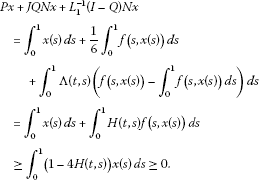Thus $\left(P+JQN+{L}_{1}^{-1}\left(I-Q\right)N\right)\left(K\right)\subset K$.

Let

${\mathrm{\Omega }}_{3}=\left\{x\in X:\parallel x\parallel

Clearly, ${\mathrm{\Omega }}_{3}$ and ${\mathrm{\Omega }}_{4}$ are bounded and open sets and

$\theta \in {\mathrm{\Omega }}_{3}\subset {\overline{\mathrm{\Omega }}}_{3}\subset {\mathrm{\Omega }}_{4}.$

Next, we show that

(3.5)

On the contrary, suppose that there exists ${x}_{5}\in \partial {\mathrm{\Omega }}_{4}\cap K$ such that

$\left(P+JQN\right){x}_{5}+{L}_{1}^{-1}\left(I-Q\right)N{x}_{5}\ge {x}_{5}.$

Then

$-{x}_{5}^{″}\left(t\right)\le f\left(t,{x}_{5}\left(t\right)\right),\phantom{\rule{1em}{0ex}}t\in \left[0,1\right].$

Let ${t}_{2}\in \left[0,1\right]$ such that ${x}_{5}\left({t}_{2}\right)={max}_{t\in \left[0,1\right]}{x}_{5}\left(t\right)=b$. Using the boundary conditions, we have ${t}_{2}\in \left(0,1\right)$. In this case, ${x}_{5}^{\prime }\left({t}_{2}\right)=0$, ${x}_{5}^{″}\left({t}_{2}\right)\le 0$. This gives

$0\le -{x}_{5}^{″}\left({t}_{2}\right)\le f\left({t}_{2},{x}_{5}\left({t}_{2}\right)\right)=f\left({t}_{2},b\right),$

which is a contradiction to condition (H1). Therefore (3.5) holds.

Finally, similar to the proof of (3.3), it follows from condition (H2) that

Consequently all conditions of Theorem 2.1 are satisfied. Therefore, there exists ${x}^{\ast }\in K\cap \left({\overline{\mathrm{\Omega }}}_{4}\mathrm{\setminus }{\mathrm{\Omega }}_{3}\right)$ such that $L{x}^{\ast }=N{x}^{\ast }$ with ${x}^{\ast }\in K$ and $a\le \parallel {x}^{\ast }\parallel \le b$ and the assertion follows. □

## References

1. Cremins CT: A fixed-point index and existence theorems for semilinear equations in cones. Nonlinear Anal. 2001, 46: 789-806. 10.1016/S0362-546X(00)00144-9

2. Feckan M: Existence of nonzero nonnegative solutions of semilinear equations at resonance. Comment. Math. Univ. Carol. 1998, 39: 709-719.

3. Gaines R, Mawhin J Lecture Notes in Mathematics 568. In Coincidence Degree, and Nonlinear Differential Equations. Springer, Berlin; 1977.

4. Mawhin J: Equivalence theorems for nonlinear operator equations and coincidence degree theory for some mappings in locally convex topological vector spaces. J. Differ. Equ. 1972, 12: 610-636. 10.1016/0022-0396(72)90028-9

5. Mawhin J: Landesman-Lazer’s type problems for nonlinear equations. Conf. Semin. Mat. Univ. Bari 1977, 147: 1-22.

6. Nieto JJ: Existence of solutions in a cone for nonlinear alternative problems. Proc. Am. Math. Soc. 1985, 94: 433-436. 10.1090/S0002-9939-1985-0787888-1

7. Przeradzki B: A note on solutions of semilinear equations at resonance in a cone. Ann. Pol. Math. 1993, 58: 95-103.

8. Santanilla J: Existence of nonnegative solutions of a semilinear equation at resonance with linear growth. Proc. Am. Math. Soc. 1989, 105: 963-971. 10.1090/S0002-9939-1989-0964462-9

9. Gaines R, Santanilla J: A coincidence theorem in convex sets with applications to periodic solutions of ordinary differential equations. Rocky Mt. J. Math. 1982, 12: 669-678. 10.1216/RMJ-1982-12-4-669

10. Petryshyn WV: On the solvability of $x\in Tx+\lambda Fx$ in quasinormal cones with T and F k -set contractive. Nonlinear Anal. 1981, 5: 585-591. 10.1016/0362-546X(81)90105-X

11. Cremins CT: Existence theorems for semilinear equations in cones. J. Math. Anal. Appl. 2002, 265: 447-457. 10.1006/jmaa.2001.7746

12. Deimling K: Nonlinear Functional Analysis. Springer, Berlin; 1985.

13. Chu J, Lin X, Jiang D, O’Regan D, Agarwal RP: Multiplicity of positive solutions to second order differential equations. Bull. Aust. Math. Soc. 2006, 73: 175-182. 10.1017/S0004972700038764

14. Chu J, Torres PJ: Applications of Schauder’s fixed point theorem to singular differential equations. Bull. Lond. Math. Soc. 2007, 39: 653-660. 10.1112/blms/bdm040

15. Chu J, Nieto JJ: Impulsive periodic solutions of first-order singular differential equations. Bull. Lond. Math. Soc. 2008, 40: 143-150. 10.1112/blms/bdm110

16. Chu J, Zhang Z: Periodic solutions of singular differential equations with sign-changing potential. Bull. Aust. Math. Soc. 2010, 82: 437-445. 10.1017/S0004972710001607

17. Franco D, Webb JRL: Collisionless orbits of singular and nonsingular dynamical systems. Discrete Contin. Dyn. Syst. 2006, 15: 747-757.

18. O’Regan D, Zima M: Leggett-Williams norm-type theorems for coincidences. Arch. Math. 2006, 87: 233-244. 10.1007/s00013-006-1661-6

19. Santanilla J: Some coincidence theorems in wedges, cones and convex sets. J. Math. Anal. Appl. 1985, 105: 357-371. 10.1016/0022-247X(85)90053-8

20. Petryshyn WV: Using degree theory for densely defined A-proper maps in the solvability of semilinear equations with unbounded and noninvertible linear part. Nonlinear Anal. 1980, 4: 259-281. 10.1016/0362-546X(80)90053-X

## Acknowledgements

JC was supported by the National Natural Science Foundation of China (Grant No. 11171090, No. 11271333 and No. 11271078), the Program for New Century Excellent Talents in University (Grant No. NCET-10-0325), China Postdoctoral Science Foundation funded project (Grant No. 2012T50431). FW was supported by the National Natural Science Foundation of China (Grant No. 10971179) and the Natural Science Foundation of Changzhou University (Grant No. JS201008).

## Author information

Authors

### Corresponding author

Correspondence to Jifeng Chu.

### Competing interests

The authors declare that they have no competing interests.

### Authors’ contributions

Both authors read and approved the final manuscript.

## Rights and permissions

Reprints and Permissions

Chu, J., Wang, F. An order-type existence theorem and applications to periodic problems. Bound Value Probl 2013, 37 (2013). https://doi.org/10.1186/1687-2770-2013-37

• Accepted:

• Published:

• DOI: https://doi.org/10.1186/1687-2770-2013-37

### Keywords

• fixed point index
• order-type existence theorem
• cone expansion and compression
• positive solutions
• periodic boundary value problems